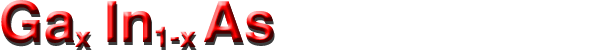## Optical properties

 Ga0.47In0.53As GaxIn1-xAs Remarks Referens Dielectric constant (static) 13.9 15.1-2.87x+0.67x2 300 K Dielectric constant (high frequency) 11.6 12.3-1.4x 300 K Infrared refractive index n 3.43 cm2 V-1s-1 (3.51-0.16x ) V-1s-1 300 K Goldberg Yu.A. & N.M. Schmidt (1999) Radiative recombination coefficient 0.96 x 10-10 cm2/s see Impact Ionization 300 K Optical phonon energy 34 meV see Raman-active phonon modes 300 K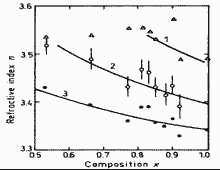Refractive index n versus alloy composition x at different photon energies 1 1.2 eV2 0.9 eV3 0.6 eV. Takagi (1978)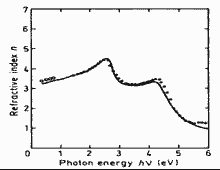Refractive index n versus photon energy for x=0.47. 300 K. Adachi (1992)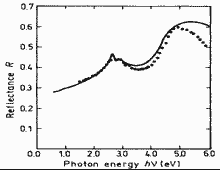Normal incidence reflectivityversus photon energy for x=0.47. 300 K. Adachi (1992)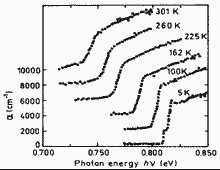The absorption coefficient versus photon energy at different temperatures for x=0.47. Electron concentration no=8·1014 cm-3. Curves are shifted vertically for clarity. Zielinski et al. (1986)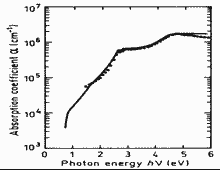The absorption coefficient versus photon energy for x=0.47, 300 K. Adachi (1992)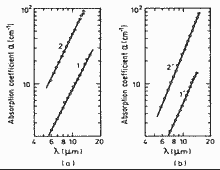Free carrier absorption coefficient versus wavelength. a - T=300 K, b - T=92 K. 1 x=0.08, Nd=1.4·1017 cm-3 2 x=0.1 Nd=5.4·1017 cm-3. Aliev et al. (1987)

A ground state Rydberg energy Rx1=2.5 meV (for x=0.47).